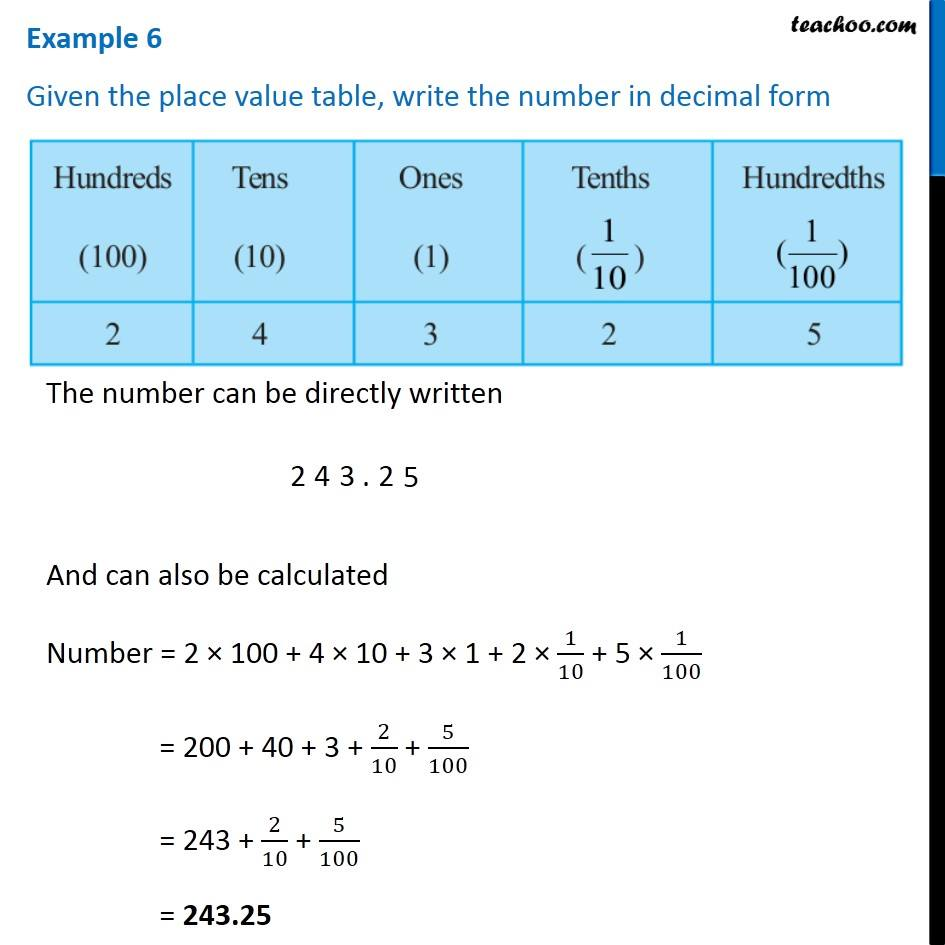Examples

Chapter 8 Class 6 Decimals
Serial order wiseLearn in your speed, with individual attention - Teachoo Maths 1-on-1 Class

### Transcript

Question 6 Given the place value table, write the number in decimal form The number can be directly written And can also be calculated Number = 2 × 100 + 4 × 10 + 3 × 1 + 2 × 1/10 + 5 × 1/100 = 200 + 40 + 3 + 2/10 + 5/100 = 243 + 2/10 + 5/100 = 243.25